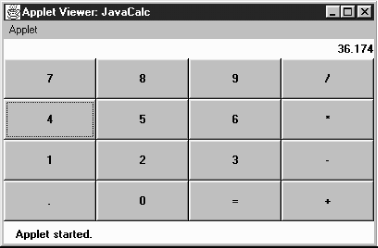home | O'Reilly's CD bookshelfs | FreeBSD | Linux | Cisco | Cisco Exam

### 5.4 A Simple Calculator

It is always helpful to see complete and somewhat useful examples after learning something new. Example 5.2 shows a working calculator that performs floating point addition, subtraction, multiplication, and division. Figure 5.4 shows the calculator in operation. The button in the lower left corner is a decimal point. This applet uses a number of classes that will be discussed later in the book (most notably, some layout managers and a Panel); try to ignore them for now. Focus on the action() and compute() methods; action() figures out which button was pressed, converting it to a digit (0-9 plus the decimal point) or an operator (=, +, -, *, /). As you build a number, it is displayed in the label lab, which conveniently serves to store the number in string form. The compute() method reads the label's text, converts it to a floating point number, does the computation, and displays the result in the label. The addButtons() method is a helper method to create a group of Button objects at one time.

#### Example 5.2: Calculator Source Code

```import java.awt.*;
import java.applet.*;
public class JavaCalc extends Applet {
Label lab;
boolean firstDigit = true;
float savedValue = 0.0f;     // Initial value
String operator = "=";  // Initial operator
public void addButtons (Panel p, String labels) {
int count = labels.length();
for (int i=0;i<count;i++)
}
public void init () {
setLayout (new BorderLayout());
add ("North", lab = new Label ("0", Label.RIGHT));
Panel p = new Panel();
p.setLayout (new GridLayout (4, 4));
}
public boolean action (Event e, Object o) {
if (e.target instanceof Button) {
String s = (String)o;
if ("0123456789.".indexOf (s) != -1) { // isDigit
if (firstDigit) {
firstDigit = false;
lab.setText (s);
} else {
lab.setText (lab.getText() + s);
}
} else { // isOperator
if (!firstDigit) {
compute (lab.getText());
firstDigit = true;
}
operator = s;
}
return true;
}
return false;
}
public void compute (String s) {
float sValue = new Float (s).floatValue();
char c = operator.charAt (0);
switch (c) {
case '=':   savedValue  = sValue;
break;
case '+':   savedValue += sValue;
break;
case '-':   savedValue -= sValue;
break;
case '*':   savedValue *= sValue;
break;
case '/':   savedValue /= sValue;
break;
}
lab.setText (String.valueOf(savedValue));
}
}
```

#### Figure 5.4: Calculator applet